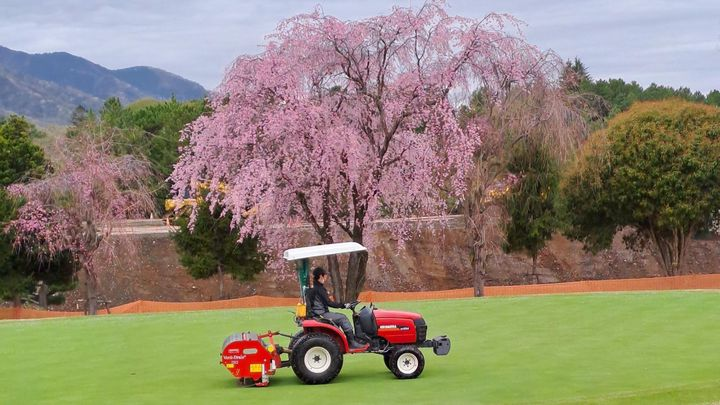# The growth ratio equationGrowth ratio shows how much the grass is growing during any season compared with expected growth at that time of year.

I’ve been recommending the growth ratio as a way to check the observed (measured) growth of the grass at any particular time of year.

The clipping volume on its own tells you how much the grass is actually growing. The growth ratio tells you something subtly different—how much the grass is growing in relation to how much it is expected to grow based on recent temperatures.

This1 is the growth ratio equation:

$\frac{ClipVol}{20(GP)} = GR$

where…

$GR$ is the turfgrass growth ratio

$ClipVol$ is the clipping volume, expressed in units of mL/m2

$20$ is the standard amount of clippings, set at 20 mL/m2

$GP$ is the temperature-based turfgrass growth potential developed by PACE Turf

If you are using a different measuring unit for clipping volume, then use the equivalent of 20 mL/m2 in the denominator, with whatever unit you are measuring in, and express your $ClipVol$ in the numerator in those same units. Now you have a ratio that immediately shows how much the grass is growing compared with the expected amount of clippings given the recent weather.

For example, if the clipping volume is 12, and the GP is 0.5, then the growth ratio would be:

$\frac{12}{20(0.5)} = 1.2$

I have usually looked at a trailing 7, 14, or 30 day average of growth ratio. See the related posts below for more about the growth ratio.

Also, see Jason Haines’—he came up with the growth ratio (or turfgrass speedo) idea—blog post titled Turfgrass speedo is still my most important tool for managing turf growth after 4 years.

1. If you read this post as an email, the equations won’t render. To see the equations, please view this post on the ATC website. ↩︎